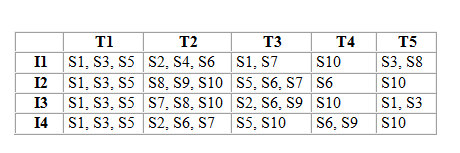# GATE | Gate IT 2005 | Question 45

A hardwired CPU uses 10 control signals S1 to S10, in various time steps T1 to T5, to implement 4 instructions I1 to I4 as shown below:Which of the following pairs of expressions represent the circuit for generating control signals S5 and S10 respectively?

((Ij+Ik)Tn indicates that the control signal should be generated in time step Tn if the instruction being executed is Ij or lk)

(A)
S5=T1+I2⋅T3 and

S10=(I1+I3)⋅T4+(I2+I4)⋅T5
(B) S5=T1+(I2+I4)⋅T3 and

S10=(I1+I3)⋅T4+(I2+I4)⋅T5
(C) S5=T1+(I2+I4)⋅T3 and

S10=(I2+I3+I4)⋅T2+(I1+I3)⋅T4+(I2+I4)⋅T5
(D) S5=T1+(I2+I4)⋅T3 and

S10=(I2+I3)⋅T2+I4⋅T3+(I1+I3)⋅T4+(I2+I4)⋅T5